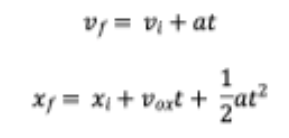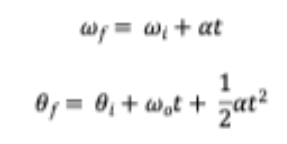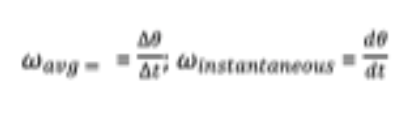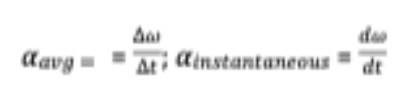# Problem: The kinematic equations (motion with constant acceleration) for motion in one dimension are:Where v is velocity and a is acceleration. For rational motion, we can define an angular velocity w and angular a, and the equation above become:More generally we have the following definitions:andUse the relationships above to derive the equations of motion for rotation with constant rotational acceleration using both algebra and calculus based approaches

###### FREE Expert Solution

For a rotational motion with constant angular acceleration, α, we have:

$\overline{){\mathbf{\alpha }}{\mathbf{=}}\frac{\mathbf{d}\mathbf{\omega }}{\mathbf{d}\mathbf{t}}}$

97% (275 ratings)###### Problem Details

The kinematic equations (motion with constant acceleration) for motion in one dimension are:Where v is velocity and a is acceleration. For rational motion, we can define an angular velocity w and angular a, and the equation above become:More generally we have the following definitions:andUse the relationships above to derive the equations of motion for rotation with constant rotational acceleration using both algebra and calculus based approaches